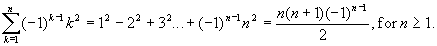## October 16th Foundation Exam

### Discrete Part I. Choose one question.

1. Prove by induction on n the following summation formula:2. Let g: A ® A be a bijective function. That is, g is both one-to-one and onto. For n ³ 2, define gn = g o g oo g , where g is composed with itself n times.

Prove that for n ³ 2, (1) gn is a bijective function from A to A; and (2) (gn)–1 = (g–1)n.

### Discrete Part II. Choose one question.

3. Let A = {a, b} denote a set of two symbols. Let S be a set of strings over A, satisfying the following property:

If u Î S and |u| > 0, then there exists a string v Î S such that u = avb.

Prove by induction that S Ì {anbn | n ³ 0}.

4. Let A = {a, b} denote a set of two symbols. Define the following two sets of strings over A:

C = {a}*{b}* , and

D = {w | w Î A*, and the number of occurrences of symbol a in w = the number of occurrences of symbol b in w}.

Prove that C Ç D = {anbn | n ³ 0}.

Solutions to the Discrete Structures questions.